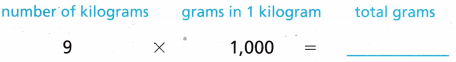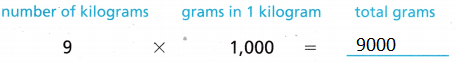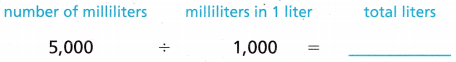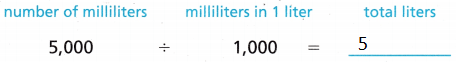# Texas Go Math Grade 4 Lesson 15.7 Answer Key Metric Units of Mass and Liquid Volume

Refer to our Texas Go Math Grade 4 Answer Key Pdf to score good marks in the exams. Test yourself by practicing the problems from Texas Go Math Grade 4 Lesson 15.7 Answer Key Metric Units of Mass and Liquid Volume.

## Texas Go Math Grade 4 Lesson 15.7 Answer Key Metric Units of Mass and Liquid Volume

Essential Question

How can you convert metric units of mass and liquid volume?1 kilogram = 1000 grams
1 liter = 1000 milliliters.

Unlock the Problem

Mass is the amoLuu of matter in an object. Metric units of mass include kilograms (kg) and grams (g). Liters (L) and milliliters (mL) are metric units of liquid volume.

The charts show the relationship between these units.Example 1 Convert larger units to smaller units.

Becky planted a flower garden full of bluebonnets. She used 9 kilograms of soil. How many grams of soil is that?So, Becky used ___________ grams of soil to plant her blue bonnets.So, Becky used 9000 grams of soil to plant her blue bonnets.

Are kilograms larger or smaller than grams?
Explanation:
1 kilogram= 1000 grams

Will the number of grams be greater than or less than the number of kilograms?
Answer: number of grams be less than the number of kilograms

What operation will you use to solve the problem?
We used multiplication to solve the problem.

Example 2 Convert smaller units to larger units.

Becky used 5,000 milliliters of water to water her bluebonnet garden. How many liters of water is that?So, Becky used __________ liters of water.So, Becky used 5 liters of water.

Math Talk

Mathematical Processes
Compare the size of a kilogram to the size of a gram. Then compare the size of a liter to the size of a milliliter.1 kilogram = 1000 grams
1 liter = 1000 milliliters.

Share and Show

Question 1.
There are 3 liters of water in a pitcher. How many milliliters of water are in the pitcher?

There are ___________ milliliters in 1 liter. Since I am changing from a larger unit to a smaller unit, I can ___________ 3 by 1,000 to find the number of milliliters in 3 liters.

So, there are ___________ milliliters of water in the pitcher.

There are 1000 milliliters in 1 liter. Since I am changing from a larger unit to a smaller unit, I can multiply 3 by 1,000 to find the number of milliliters in 3 liters.

So, there are 3000 milliliters of water in the pitcher.

Complete.

Question 2.
4 liters = ___________ milliliters
Explanation:
There are 1000 milliliters in 1 liter. Since I am changing from a larger unit to a smaller unit, I can multiply 4 by 1,000 to find the number of milliliters in 4 liters.

Question 3.
6 kilograms = ___________ grams
Explanation:
There are 1000 grams in 1 kilogram. Since I am changing from a larger unit to a smaller unit, I can multiply 6 by 1,000 to find the number of grams in 6 kilograms.

Question 4.
8,000 grams = ___________ kilograms
Explanation:
There are 1000 grams in 1 kilogram. Since I am changing from a Smaller unit to a larger unit, I can divide 8000 by 1,000 to find the number of kilograms in 8,000 grams.

Question 5.
7 liters = ___________ milliliters
Explanation:
There are 1000 milliliters in 1 liter. Since I am changing from a larger unit to a smaller unit, I can multiply 7 by 1,000 to find the number of milliliters in 7 liters.

Math Talk

Mathematical Processes
Explain how you found the number of grams in 6 kilograms in Exercise 3.
There are 1000 grams in 1 kilogram. Since I am changing from a larger unit to a smaller unit, I can multiply 6 by 1,000 to find the number of grams in 6 kilograms.

Problem Solving

Question 6.
Frank wants to fill a fish tank with 8 liters of water.
How many milliliters is that?
Explanation:
There are 1000 milliliters in 1 liter. Since I am changing from a larger unit to a smaller unit, I can multiply 8 by 1,000 to find the number of milliliters in 8 liters.

Question 7.
Kim has 3 water bottles. She fills each bottle with 1,000 milliliters of water. How many liters of water does she have?
Explanation:
There are 1000 milliliters in 1 liter.
So 3x 1000 milliliters = 3000 milliliters
Now convert to liters
Since I am changing from a Smaller unit to a larger unit, I can divide 3000 by 1,000 to find the number of liters in 3000 milliliters .

Question 8.
H.O.T. Multi-Step A 500-gram bag of granola costs $4, and a 2-kilogram bag of granola costs$15. What is the cheapest way to buy 2,000 grams of granola? Explain.
Explanation:
500-gram bag of granola costs $4. to buy 2,000 grams of granola need to buy 4 bags. Since 4 x 500 grams = 2000 grams So cost is 4 x 4 =$16
2-kilogram bag of granola costs $15. 1 kilogram is equals to 1000 grams. 2 kilograms = 2 x 1000 grams= 2000 grams to buy 2,000 grams of granola need to buy$15.

Question 9.
Sense or Nonsense? The world’s largest apple had a mass of 1,849 grams. Sue said the mass was greater than 2 kilograms. Does Sue’s statement make sense? Explain.
Answer: No. Its not make sense
Explanation:
1 kilogram = 1000 grams
2 kilogram = 2000 grams
1849 < 2000. So Sue statement is not make sense.

Unlock the Problem

Question 10.H.O.T. Apply Multi-Step Lori bought 600 grams of cayenne pepper and 2 kilograms of black pepper. How many grams of pepper did she buy?
Explanation:
1 kilogram = 1000 grams
2 kilogram = 2000 grams
600 grams of cayenne pepper and 2 kilograms of black pepper = 600 grams + 2000 grams = 2600 grams

a. What are you asked to find?
Answer: How many grams of pepper  she bought in all.

b. What information will you use?
Answer: Lori bought 600 grams of cayenne pepper and 2 kilograms of black pepper

c. Tell how you might solve th€ problem.
Answer: Changing from a larger unit to a smaller unit

d. Show how you solved the problem.
1 kilogram = 1000 grams
2 kilogram = 2000 grams
600 grams of cayenne pepper and 2 kilograms of black pepper = 600 grams + 2000 grams = 2600 grams

e. Complete the sentences.
Lori bought __________ grams of cayenne pepper.
She bought __________ grains of black pepper.
__________ + __________ = __________ grams
So, Lori bought __________ grams of pepper in all.
Lori bought 600 grams of cayenne pepper.
She bought 2000 grams grains of black pepper.
600 + 2000 = 2600 grams
So, Lori bought 2600 grams of pepper in all.

Question 11.
The largest natural pearl in the world has a mass of about 6,000 grams. What is the mass of the pearl in kilograms?
(A) 600 kilograms
(B) 6 kilograms
(C) 60,000 kilograms
(D) 60 kilograms
Explanation:
There are 6000 grams in 1 kilogram. Since I am changing from a Smaller unit to a larger unit, I can divide 6000 by 1,000 to find the number of kilograms in 6,000 grams.

Question 12.
Jen puts 10 liters of water in her aquarium. How many milliliters of water does she put in the aquarium?
(A) 100,000 milliliters
(B) 1,000 milliliters
(C) 100 milliliters
(D) 10,000 milliliters
Explanation:
There are 1000 milliliters in 1 liter. Since I am changing from a larger unit to a smaller unit, I can multiply 10 by 1,000 to find the number of milliliters in 10 liters.

Question 13.
Multi-Step Terry buys 3 kilograms of peanuts for a baseball game. He puts the peanuts in bags of 200 grams each. How many bags of peanuts does he have?
(A) 3,000 bags
(B) 150 bags
(C) 15 bags
(D) 50 bags
Explanation:
There are 1000 grams in 1 kilogram. Since I am changing from a larger unit to a smaller unit, I can multiply 3 by 1,000 to find the number of grams in 3 kilograms.
So, it is  3000 grams.
He puts the peanuts in bags of 200 grams each. 3000 ÷ 200 = 15

Texas Test Prep

Question 14.
Caroline bought a bag of onions that was labeled 5 kilograms. She needs to know how many grams that is for her recipe. How many grams is 5 kilograms?
(A) 5,000 grams
(B) 50 grams
(C) 50,000 grams
(D) 500 grams
Explanation:
There are 1000 grams in 1 kilogram. Since I am changing from a larger unit to a smaller unit, I can multiply 5 by 1,000 to find the number of grams in 5 kilograms.
So, it is  5000 grams.

### Texas Go Math Grade 4 Lesson 15.6 Homework and Practice Answer Key

Complete.Question 1.
5,000 grams = ___________ kilograms
Explanation:
There are 5000 grams in 1 kilogram. Since I am changing from a Smaller unit to a larger unit, I can divide 5000 by 1,000 to find the number of kilograms in 5,000 grams.

Question 2.
3 liters = ___________ milliliters
Explanation:
There are 1000 milliliters in 1 liter. Since I am changing from a larger unit to a smaller unit, I can multiply 3 by 1,000 to find the number of milliliters in 3 liters.

Question 3.
9 kilograms = ___________ grams
Explanation:
There are 1000 grams in 1 kilogram. Since I am changing from a Smaller unit to a larger unit, I can divide 9000 by 1,000 to find the number of kilograms in 8,000 grams.

Question 4.
6,000 milliliters = ___________ liters
Explanation:
There are 1000 milliliters in 1 liter. Since I am changing from a Smaller unit to a larger unit, I can divide 6000 by 1,000 to find the number of liters in 6000  milliliters .
So, Answer is  6 liters .

Problem Solving

Question 5.
A bucket holds 10 liters of water. How many milliliters of water does it hold?
Explanation:
There are 1000 milliliters in 1 liter. Since I am changing from a larger unit to a smaller unit, I can multiply 10 by 1,000 to find the number of milliliters in 10 liters.

Question 6.
Jessie’s cat has a mass of 5 kilograms. How many grams is Jessie’s cat?
Explanation:
There are 1000 grams in 1 kilogram. Since I am changing from a larger unit to a smaller unit, I can multiply 5 by 1,000 to find the number of grams in 5 kilograms.
So, it is  5000 grams.

Question 7.
Austin bought 2,500 grams of red apples and 3,500 grams of green apples. How many kilograms of apples did Austin buy?
Explanation:
2500 + 3500 = 6000
There are 1000 grams in 1 kilogram. Since I am changing from a Smaller unit to a larger unit, I can divide 6000 by 1,000 to find the number of kilograms in 6000 grams.

Question 8.
Kendal has a 5 liter bottle of orange juice and a 3 liter bottle of grapefruit juice. How many more milliliters of orange juice than grapefruit juice does she have?
Explanation:
There are 1000 milliliters in 1 liter. Since I am changing from a larger unit to a smaller unit, I can multiply 5 by 1,000 to find the number of milliliters in 5 liters.
So, it is 5,000 milliliters orange juice.
There are 1000 milliliters in 1 liter. Since I am changing from a larger unit to a smaller unit, I can multiply 3 by 1,000 to find the number of milliliters in 3 liters.
So, it is 3,000 milliliters orange juice.
5000 – 3000 = 2000 milliliters
Question 9.
Kent’s backpack has a mass of 6 kilograms. Brent s backpack has a mass of 8 kilograms. How many grams greater is the mass of Brent’s backpack?
Explanation:
Kent’s backpack has a mass of 6 kilograms. 6 x 100 grams = 6000 grams
Brent s backpack has a mass of 8 kilograms. 8 x 1000 grams = 8000 grams
8000 – 6000 = 2000 grams

Question 10.
A bottle can hold 2 liters of water. The bottle has 500 milliliters of water in it. How many ’ milliliters more can the bottle hold?
Explanation:
A bottle can hold 2 liters of water = 2 x 1000 = 2000 milliliters
The bottle has 500 milliliters of water in it.
2000 – 500 =1500 milliliters

Question 11.
The maximum amount a picture hook can hold is 5 kilograms. Can Jamie hang a picture that has a mass of 4,600 grams on the hook? Explain.
Explanation:
The maximum amount a picture hook can hold is 5 kilograms. 5 x 1000=5000 grams

5000 > 4600.

Lesson Check

Question 12.
A punch bowl holds 16 liters, How many milliliters does the punch bowl hold?
(A) 1,600 milliliters
(B) 160 milliliters
(C) 16,000 milliliters
(D) 160,000 milliliters
Explanation:
There are 1000 milliliters in 1 liter. Since I am changing from a larger unit to a smaller unit, I can multiply 16 by 1,000 to find the number of milliliters in 16 liters.

Question 13.
Tim bought a block of cheese that is labeled 4 kilograms. He wants to cut the cheese into blocks that weigh 200 grams. How? many blocks of cheese can Tim cut?
(A) 20
(B) 80
(C) 8
(D) 200
Explanation:
Tim bought a block of cheese that is labeled 4 kilograms. 4 x 1000 = 4000 grams
4000 ÷ 200 =80

Question 14.
Allen bought a 3-kilogram squash and a 2-kilogram squash. How many grams of squ ash did Allen buy in all?
(A) 50,000 grams
(B) 500 grams
(C) 50 grams
(D) 5,000 grams
Explanation:
1 kilogram = 1000 grams
3-kilogram squash and a 2-kilogram squash = 3 x 1000 + 2 x 1000 = 5000 grams

Question 15.
How many 25 milliliter doses of plant food can Tim get from a 1-liter bottle of plant food?
(A) 40
(B) 4
(C) 400
(D) 4,000
Explanation:
1-liter = 1000 milliliters
1000 ÷25 =40

Question 16.
Multi-Step Maria has a 2-liter bottle of juice. She pours two 300 milliliter glasses of juice. How much juice is left in the bottle?
(A) 1,940 milliliters
(B) 1,400 milliliters
(C) 800 milliliters
(D) 1,700 milliliters
Explanation:
Maria has a 2-liter bottle of juice. 2 x 1000 =2000
She pours two 300 milliliter glasses of juice = 2 x 300 =600
2000 – 600 = 1400 milliliters
Question 17.
Multi-Step Hannah bought a bag of pears that has a mass of 5 kilograms. She used 1,600 grams of pears for a recipe. What is the mass of the pears she has left?
(A) 3,400 grams
(B) 34 kilograms
(C) 3,400 kilograms
(D) 340 grams